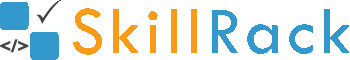Half Pyramid Pattern Printing2 months ago

The program must accept an integer N as the input. The program must print the desired pattern as shown in the Example Input/Output section.

Boundary Condition(s):
1 <= N <= 100

Input Format:
The first line contains N.

Output Format:
The first N lines containing the desired pattern as shown in the Example Input/Output section.

Example Input/Output 1:
Input:
5

Output:
1
1 2
1 2 3
1 2 3 4
1 2 3 4 5

Example Input/Output 2:
Input:
6

Output:
1
1 2
1 2 3
1 2 3 4
1 2 3 4 5
1 2 3 4 5 6

The below video explains the logic and the implementation in C programming language to print the half pyramid pattern for a given value of N.

#include <stdio.h>
#include <stdlib.h>

int main()
{
int N;
scanf("%d",&N);
for(int row=1; row<=N; row++)
{
for(int val=1; val<=row; val++)
{
printf("%d ", val);
}
printf("\n");
}
return 0;
}

#include <iostream>

using namespace std;

int main()
{
int N;
cin >> N;
for(int row = 1; row <= N; row++)
{
for(int val = 1; val <= row; val++)
{
cout << val << " ";
}
cout << endl;
}
}

import java.util.Scanner;

public class Hello {

public static void main(String[] args) {
Scanner sc = new Scanner(System.in);
int N = sc.nextInt();
for (int row = 1; row <= N; row++) {
for (int val = 1; val <= row; val++) {
System.out.print(val + " ");
}
System.out.println();
}
}
}

N = int(input())
for row in range(1,N+1):
for val in range(1,row+1):
print(val,end = " ")
print()